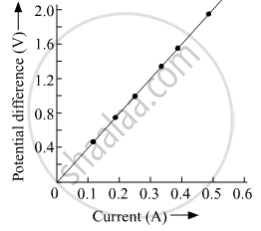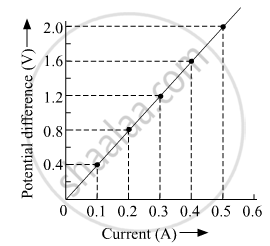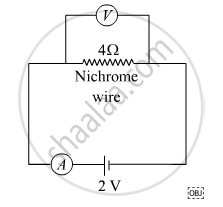# A V-i Graph for a Nichrome Wire is Given Below. What Do You Infer from this Graph? Draw a Labelled Circuit Diagram to Obtain Such a Graph. - Science

Graph

A V-I graph for a nichrome wire is given below. What do you infer from this graph? Draw a labelled circuit diagram to obtain such a graph.#### SolutionThe graph between V & I  is a straight line.

So we can conclude that  Current flowing through the wire is directly proportional to the potential difference V across it.
The resistance of the wire can be calculated as

"R" = "v"/1 = 0.4/0.1 = 4Ω

We can also conclude that nichrome wire has a constant value of the resistance as 4 Ω4 Ω.

nichrome wire follows ohm's law thus it behaves as an ohmic conductor.

The circuit diagram corresponding to the above graph is as shown below.Concept: Electric Potential (Electrostatic Potential) and Potential Difference
Is there an error in this question or solution?